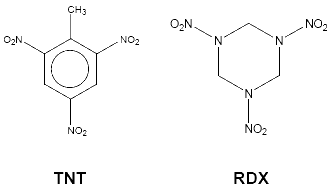# ICHO Past Year Question Papers 2011

### This question is about a popular snack food.

The ingredients of a ‘salt and vinegar flavour baked corn snack’ are listed as:

a. Maize

b. Vegetable oil

c. FlavouringSalt

A small bag typically contains 22 g of the snack.

The snack was analysed as follows:

## Calorimetry

A 2.0 g sample of the snack was burnt completely. The temperature of the water in the calorimeter rose by 20.9 oC. The calorimeter contained 500 g of water.

## Titration

The crushed contents of a whole small bag of the snack were washed with distilled water to dissolve all of the salt. The resulting solution was made up to 250 cm3 in a volumetric flask.

A 25.0 cm3 portion of this solution was titrated against 0.100 mol dm-3 aqueous silver nitrate(V) using potassium chromate(VI) as the indicator. 8.20 cm3 of aqueous silver nitrate(V) solution were required to reach the red-brown end point.

The specific heat capacity of water is 4.18 J K-1 g-1 1kJ ≡ 0.239 dietary calories:

a) Calculate the number of dietary calories in a typical small bag of the snack.

b) Give the chemical formula for (i) silver nitrate(V) and (ii) potassium chromate(VI).

c) Write an ionic equation for the titration reaction and calculate the mass of sodi chloride in a typical small bag of the snack. The ‘sharp’ taste of the snack comes from citric acid. The IUPAC recommended name for citric acid is 2-hydroxypropane-1,2,3-tricarboxylic acid.

d) Draw the skeletal structure for citric acid.  A carbon atom bonded to four different groups is called a chiral centre or an asymmetric carbon atom.

e) How many chiral centres does a molecule of citric acid contain?

This question is about calcium carbide:

Calcium carbide, CaC2, also known as calcium acetylide, is produced in large quantities around the world, mostly in the Far East. Calcium carbide has been used in a variety of ways, including as a component in self-igniting maritime distress flares.

Use the following information about calcium carbide to help you answer questions (a)(g) below.

• Calcium carbide is formed from the reaction between lime (calcium oxide) and coke (carbon) at about 2000 oC. The side-product of this reaction is a toxic gas, A, that is a reducing agent.

• The lime used in the production process commonly contains a small amount of calcium phosphate(V) as an impurity. In the reaction with coke, this impurity reacts to form compound B (a simple ionic compound of calcium and phosphorus) as well as gas A.

• Calcium carbide reacts with excess water to form a colourless, flammable gas, C. A white solid is also formed which is slightly soluble in water to give a colourless alkaline solution. Gas C is a hydrocarbon containing 92.3% carbon by mass. The relative molecular mass of C is shown to be 26.

• The addition of calcium carbide to water generates an unpleasant smell. It is now known that it is actually the reaction of water with compound B that produces this smell.

a) Draw a ‘dot-cross’ diagram of the carbide ion, C22-.

b) Which two well-known diatomic gases are iso-electronic (same number of electrons) with the carbide ion?

c) Write a balanced equation for the formation of calcium carbide and gas A from lime and coke.

d) Calculate (i) the empirical formula and (ii) the molecular formula of hydrocarbon C.

e) Write a balanced equation for the reaction of calcium carbide with water.

f) Write a balanced equation for the reaction between calcium phosphate(V) and coke to form gas A and compound B.

g) Suggest the identity of the molecule responsible for the unpleasant odour that is produced when compound B reacts with water.

This question is about explosives:

Explosives are often associated with causing destruction and harm, but explosives have also played major role in civil engineering for hundreds of years. ‘Blasters’ (explosives engineers) need a good understanding of geology and the science of explosives to be able to design explosions ‘to order’.Explosions occur when reactions proceed so that heat is generated more rapidly than it can be dispersed.

Some high explosives have the general formula CaHbNcOd. The oxidiser (O) and the fuel (C, H) are present in the same molecule. Nitrogen atoms are present so that there is the highly exothermic production of hot N2 gas.

a) Find an expression for d (in terms of a, b and c) that must be satisfied for an explosive CaHbNcOd to decompose to form CO2, H2O and N2 only.

The oxygen balance of an explosive is the mass of oxygen either in excess (i.e. the balance is positive) or in deficit (i.e. the balance is negative) of that required for complete oxidation of C and H. The oxygen balance is expressed as a percentage of the molar mass of the explosive.

b) Write a general expression for the oxygen balance of CaHbNcOd.

c) Calculate the oxygen balance for RDX, whose structure is given above. When the explosive does not contain enough oxygen, there is incomplete oxidation of C and/or H.

In the case of nitrated aromatic compounds, the reaction products are given by the following set of ‘rules’:

* Carbon atoms react with the available oxygen to form CO

* N atoms combine to form N2

* 1/3 of the CO produced is converted to C+CO2

* 1/6 of the original CO produced reacts with any available H in the compound to form C + H2O

* Write the equation for the explosion of TNT.

* Calculate the mass of TNT that will explode to produce 1.0 dm3 of gas at room Calculate the mass of TNT that will explode to produce 1.0 dm3 of gas at room temperature and pressure. [The molar volume of any gas = 24 dm3 at RTP]### Course Features

• Video Lectures
• Revision Notes
• Previous Year Papers
• Mind Map
• Study Planner
• NCERT Solutions
• Discussion Forum
• Test paper with Video Solution